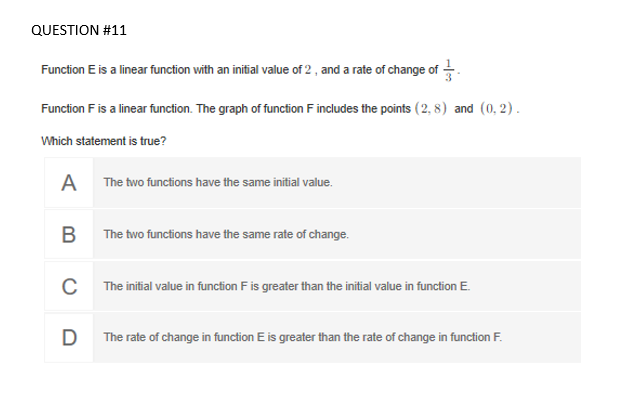# Function E is a linear function with an initial value of 2 , and a rate of change of . Function F is a linear function. The graph of function F includes the points (2, 8) and (0, 2). Which statement is true? A The two functions have the same initial value. B The two functions have the same rate of change. C The initial value in function F is greater than the initial value in function E. D The rate of change in function E is greater than the rate of change in function F.

Question

Reposting.  This was all the infor given in the question.help_outlineImage TranscriptioncloseFunction E is a linear function with an initial value of 2 , and a rate of change of . Function F is a linear function. The graph of function F includes the points (2, 8) and (0, 2). Which statement is true? A The two functions have the same initial value. B The two functions have the same rate of change. C The initial value in function F is greater than the initial value in function E. D The rate of change in function E is greater than the rate of change in function F. fullscreen

1 Rating

### Want to see this answer and more?

Experts are waiting 24/7 to provide step-by-step solutions in as fast as 30 minutes!*

*Response times may vary by subject and question complexity. Median response time is 34 minutes for paid subscribers and may be longer for promotional offers.
Tagged in
Math
Algebra